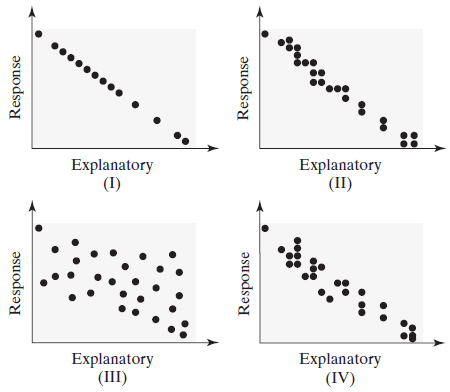×
Get Full Access to Statistics: Informed Decisions Using Data - 5 Edition - Chapter 4.1 - Problem 14
Get Full Access to Statistics: Informed Decisions Using Data - 5 Edition - Chapter 4.1 - Problem 14

×

# ?Match the linear correlation coefficient to the scatter diagram. The scales on the x- and y-axes are the same for each diagram. (a) r = -0.969ISBN: 9780134133539 240

## Solution for problem 14 Chapter 4.1

Statistics: Informed Decisions Using Data | 5th Edition

• Textbook Solutions
• 2901 Step-by-step solutions solved by professors and subject experts
• Get 24/7 help from StudySoup virtual teaching assistantsStatistics: Informed Decisions Using Data | 5th Edition

4 5 1 272 Reviews
25
2
Problem 14

Match the linear correlation coefficient to the scatter diagram. The scales on the x- and y-axes are the same for each diagram.(a) r = -0.969

(b) r = -0.049

(c) r = -1

(d) r = -0.992

Step-by-Step Solution:

Step 1 of 5) Match the linear correlation coefficient to the scatter diagram. The scales on the x- and y-axes are the same for each diagram. Using Technology In Minitab, there is a column of numbers left of the stem. The (6) indicates that there are 6 observations in the class containing the middle value (called the median). The values above (6) represent the number of observations less than or equal to the upper-class limit of the class. For example, 11 states have percentages in poverty less than or equal to 10.9. The values in the left column below (6) indicate the number of observations greater than or equal to the lower class limit of the class. For example, 19 states have percentages in poverty greater than or equal to 15.0. In Other Words, Using split stems is like adding more classes to a frequency distribution. Now Work Problem 45.

Step 2 of 2

## Discover and learn what students are asking

Statistics: Informed Decisions Using Data : Scatter Diagrams and Correlation
?The _______ variable is the variable whose value can be explained by the value of the explanatory variable.

Unlock Textbook Solution